Thevenin Equivalent and Norton Equivalent Circuits

In the Open Circuit and Short Circuit section we talked about looking "into" two terminals of a circuit, treating the circuit otherwise as an unknown black box. This pair of two terminals is sometimes called one port.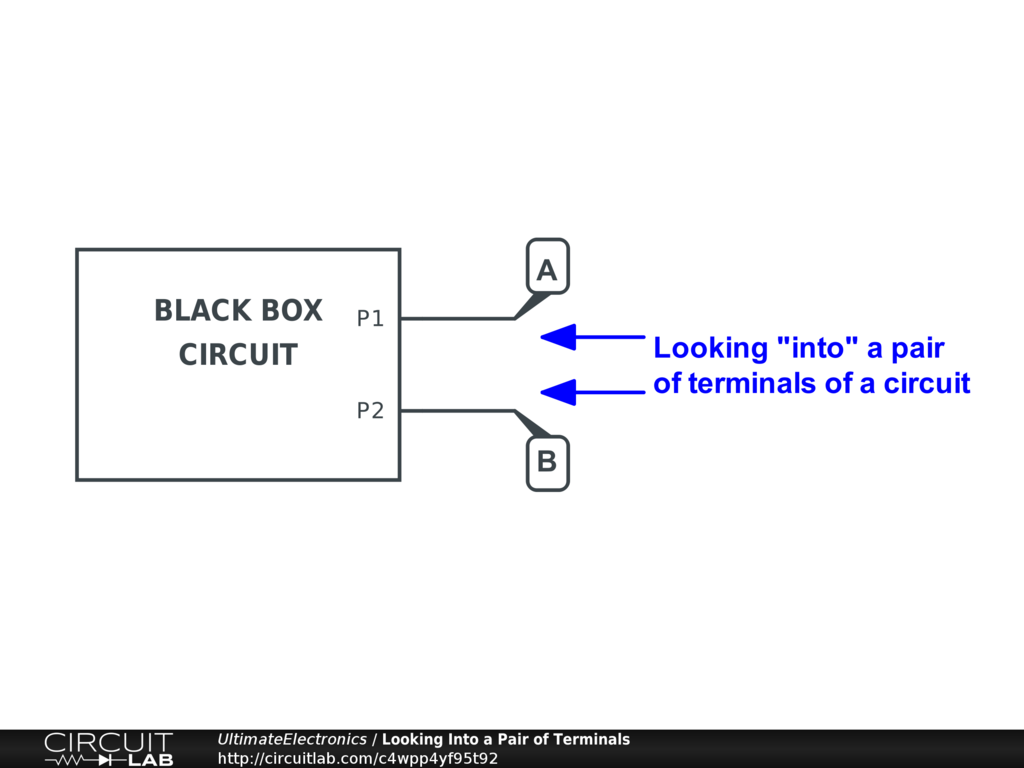In that section, we briefly conisdered what would happen if we applied two extreme conditions to these outside terminals:

• When open circuit, there would be some possibly nonzero voltage across the two terminals, but always zero current flow.
• When short circuit, there would be some possibly nonzero current, but always zero voltage difference.

These are two points on a V-I curve that we could trace out by looking into any pair of terminals of a circuit.

If the circuit inside our black box is a linear circuit composed only of voltage sources, current sources, and resistors, then the entire system of equations is completely linear. (If not, it can still be approximated by a linearized model.)

As the system is linear, we can connect our two V-I curve points with a straight line. This line will have some open circuit voltage $\text{OCV}$ at zero current, and will then have some voltage slope as increasing current is drawn. Eventually, for some value of current, the terminal voltage difference reaches zero; this current is the short-circuit current $\text{SCC}$. The (negative) slope of this line is the equivalent resistance of the arrangement:

$$R_{\text{eq}} = \frac {\text{OCV}} {\text{SCC}}$$

Because the system is linear, we can consider, for example, connecting a current source to the two external terminals. Click the schematic to enlarge: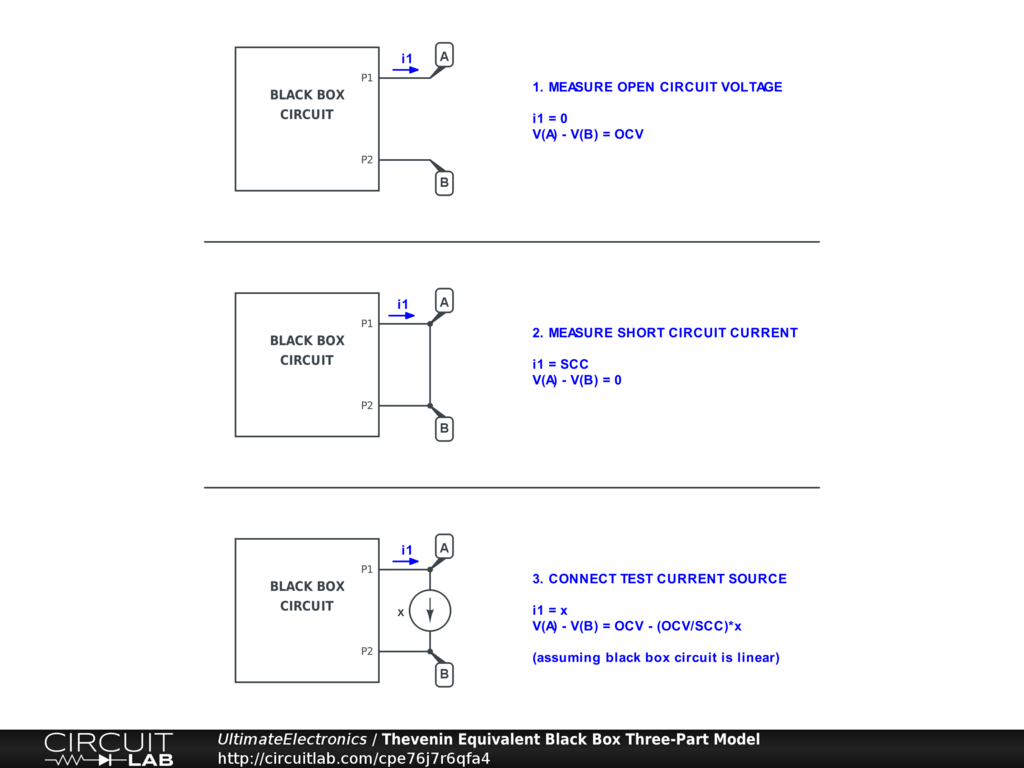1. If we set the current to 0, the voltage across the two terminals will be $$V_{A B} (i=0) = \text{OCV}$$
2. If we set the current to $\text{SCC}$, the voltage across the two terminals will be zero: $$V_{A B} (i=\text{SCC}) = 0$$
3. If we set the current to any other value $x$, the voltage across the two terminals will be along the straight line connecting those two points on the V-I plane: \begin{align} V_{A B} (i=x) & = \text{OCV} \big( 1 - \frac {1} {\text{SCC}} x\big) \\ V_{A B} (i=x) & = \text{OCV} - \frac {\text{OCV}} {\text{SCC}} x \end{align}

Note that this last equation is the same as the equation produced by the following circuit with just one voltage source and one resistor: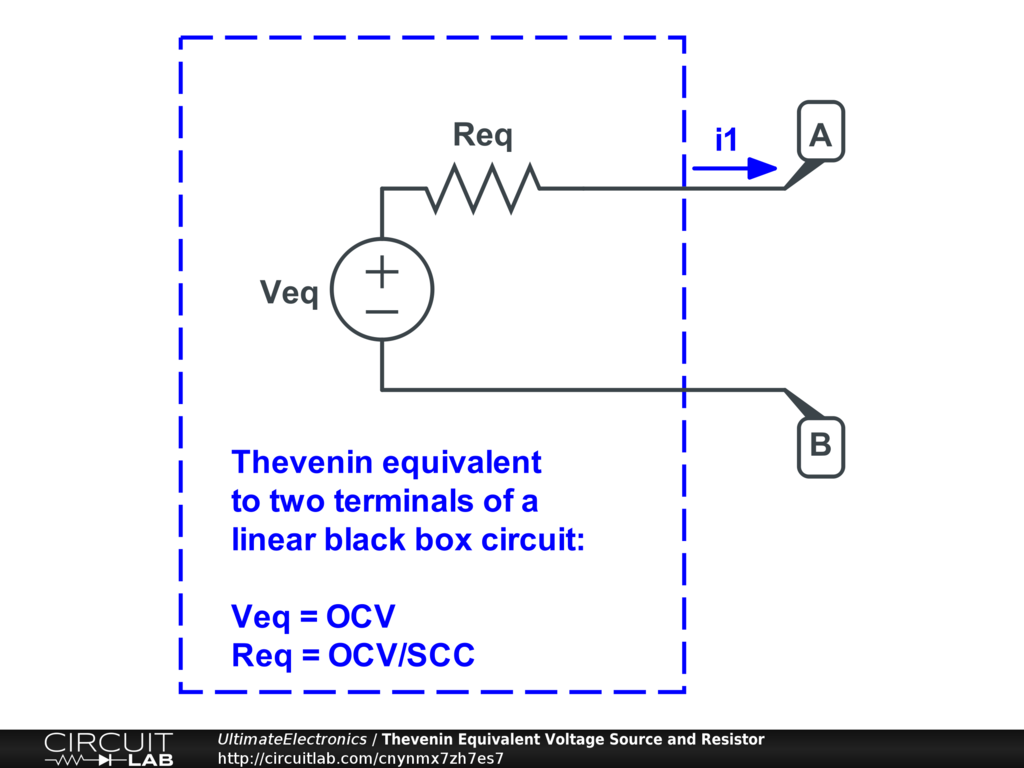The voltage source and resistor have the values:

\begin{align} V_{\text{eq}} & = \text{OCV} \\ R_{\text{eq}} & = \frac {\text{OCV}} {\text{SCC}} \end{align}

This simplified circuit with just one voltage source and one resistor is the Thevenin equivalent circuit. The key idea is that from the perspective of outside of the black box, the behavior of this equivalent circuit to the black box circuit at those two terminals is absolutely identical. We can demonstrate this equivalence by connecting our test current source again: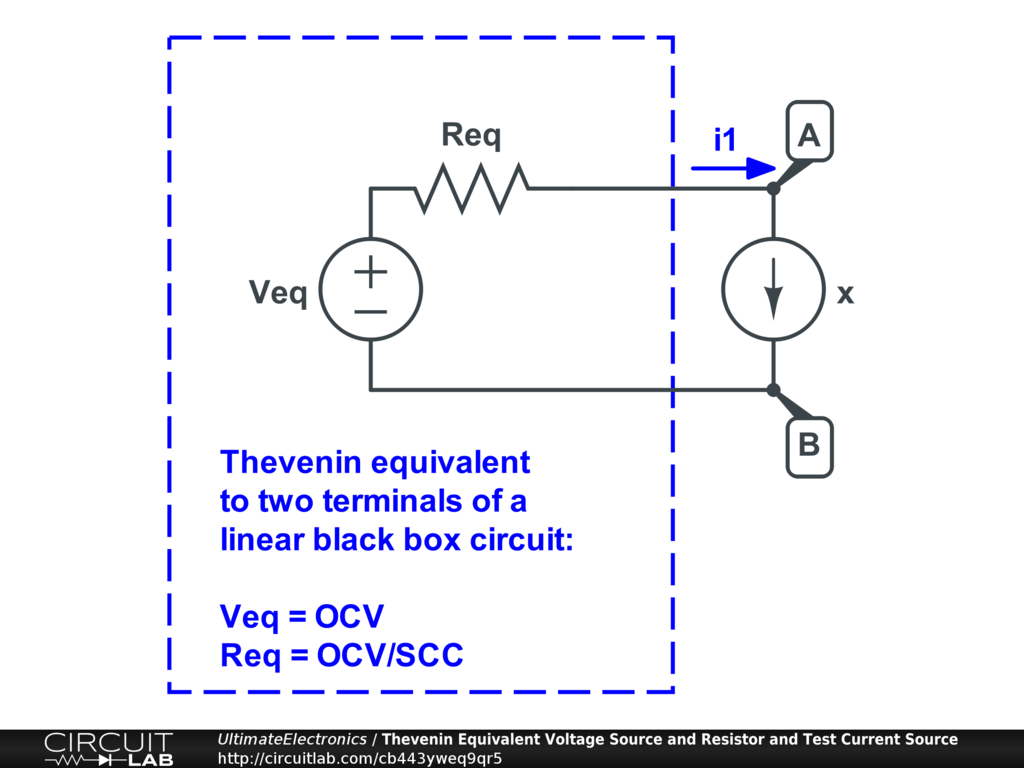1. If we set the test current $x=0$, there is no voltage drop across the resistor, so: $$V_{A B} (i=0) = V_{\text{eq}} = \text{OCV}$$
2. If we set the test current $x=\text{SCC}$, the resistor will have a voltage drop $R_{\text{eq}} \cdot \text{SCC} = \frac {\text{OCV}} {\text{SCC}} \cdot \text{SCC} = \text{OCV}$, which exactly cancels out the voltage $V_\text{eq}$, so: $$V_{A B} (i=\text{SCC}) = 0$$
3. If we set the test current to some other value $x$, the resistor will have a voltage drop $R_{\text{eq}} \cdot x = \frac {\text{OCV}} {\text{SCC}} x$, so: $$V_{A B} (i=x) = \text{OCV} - \frac {\text{OCV}} {\text{SCC}} x$$

These I-V curve values are identical to that for the unknown black box system above, even though we've simplified an unknown and possibly very complicated network into just a single voltage source and a single resistor.

Of course, internal to the black box, this neglects other possibly nonlinear effects, such as a resistor overheating. But if we assume a purely linear circuit, or a linearized model of a nonlinear circuit, then this equivalent circuit performs the same and is much easier to think about and analyze.

Being able to make a simplified linear model of a pair of terminals is incredibly useful, especially once we start connecting multiple subsystems together. For example, in a power supply, we can examine how much voltage will drop as we increase the current load. Or in an amplifier, we can find out how much one amplifier stage's output will be reduced because of loading from driving the input of the next stage. By simplifying these problems into the simplest possible form -- often just a single equivalent resistance value that we call input impedance or output impedance -- we can rapidly determine these effects without considering the entire system within.

Finding the Thevenin Equivalent Circuit by Hand

Starting from a linear circuit, finding a Thevenin equivalent circuit by hand is usually quite simple:

1. Identify your pair of nodes. You can choose any two distinct circuit nodes (or terminals) as your two nodes.
2. Measure the open circuit voltage. Solve the circuit and find the voltage difference between those nodes.
3. Add a test current source. Connect a new current source between the two nodes.
4. Find the change in voltage as you vary the test current. Use superposition to make this easy to solve. The slope of this V-I line is the equivalent resistance.
5. Construct the equivalent voltage source and equivalent resistor. Use the open circuit voltage from step 2, and the equivalent resistance from step 4, to construct the Thevenin equivalent circuit.

Let's apply these steps to a circuit we first saw in Solving Circuit Systems and again in Superposition: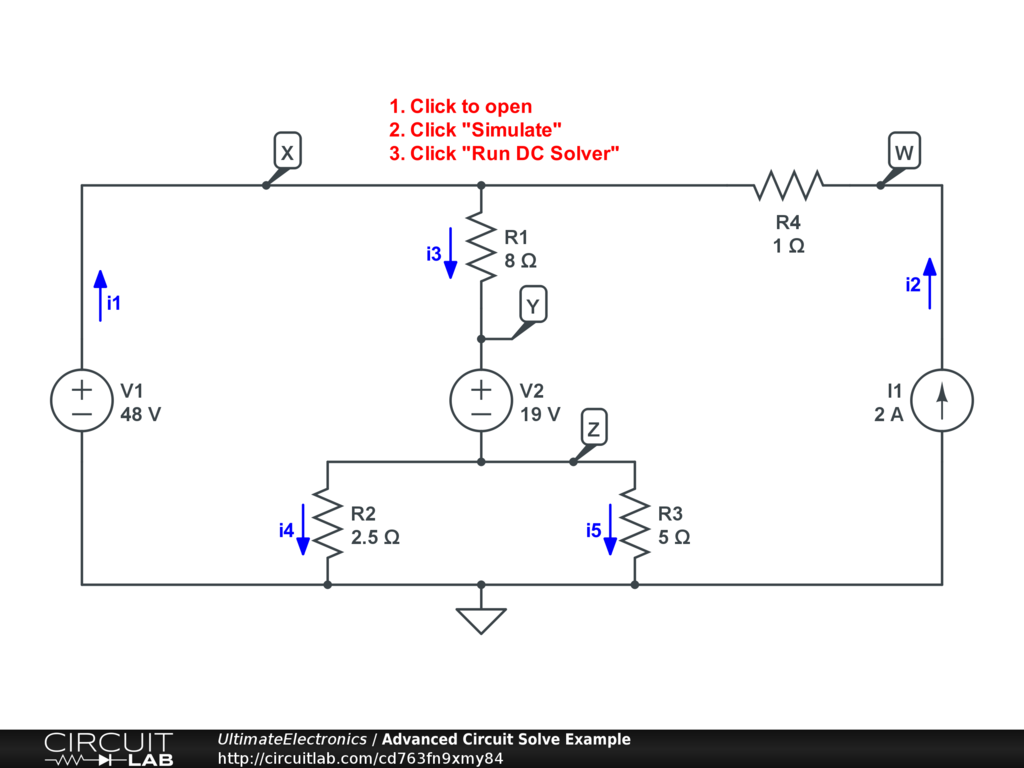For one circuit like the one above, we can construct multiple different Thevenin equivalent circuits because we can choose which pair of nodes to look at. In practice, you'll pick which nodes to use based on your application: for example, if you're looking at an amplifier or a power supply, you're probably most interested in the input-to-ground and output-to-ground pairs of terminals. Note that the Thevenin equivalent circuits we find looking into different pairs of terminals will be very different from each other!

Let's take the circuit above and approach it from a few sets of terminals separately.

For nodes W and ground:

We'll start by exposing terminals W and ground as our two terminals. First, we'll just draw a dashed rectangle to indicate what we'll be making an equivalent circuit of (our "black box"), and we'll add an external test current source Itest: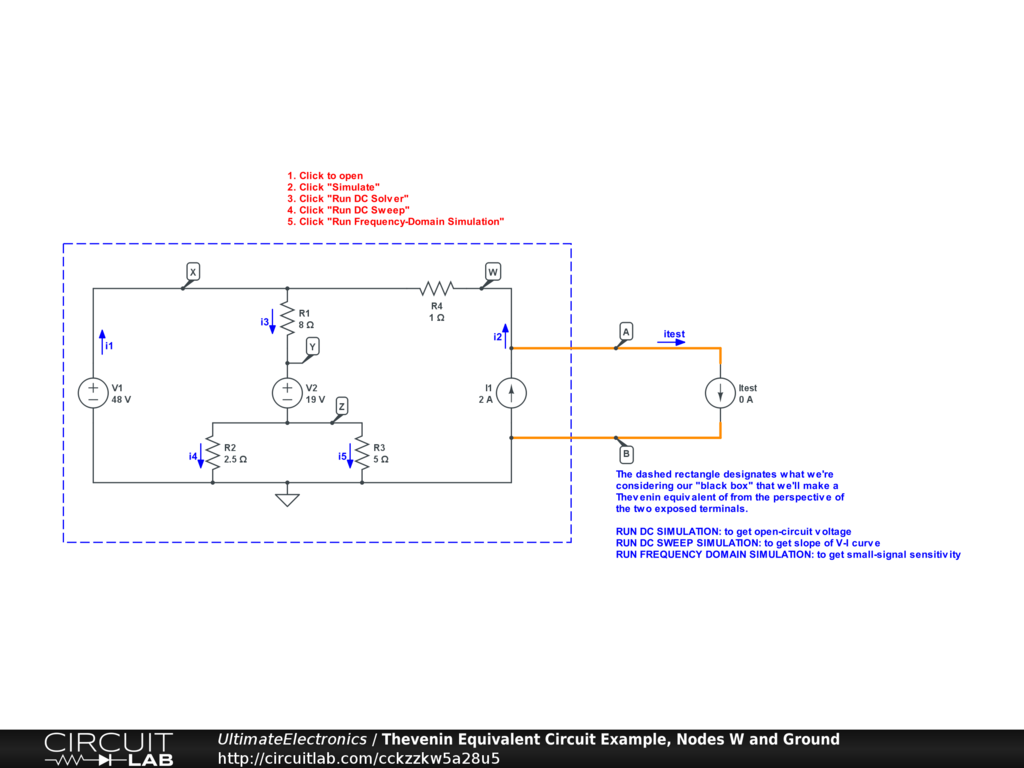Interactive Exercise We can solve this circuit quickly through simulation, and if you click to open the circuit, you'll see how we've already set up the simulation modes to quickly find the Thevenin equivalent values.

But for now, let's solve this by hand through superposition:

For step 1: we've identified the internal nodes W and ground, and have labeled them again A and B as equivalent node names.

For step 2: we've already solved this circuit in the Solving Circuit Systems and found that $V_W = 50$, so:

$$V_{\text{eq}} = 50 \ \text{V}$$

For step 3: we've added the current source Itest.

For step 4: we can use superposition because we're only interested in the contribution of the current source Itest and how it effects the terminal voltages of interest. We've effectively modeled the other (internal) sources in step 2, and now we're simply adding one more source Itest.

Let's turn off all of the internal sources by setting voltages V1 and V2 to zero (making each become a short circuit) and setting current source I1 to zero (making it an open circuit):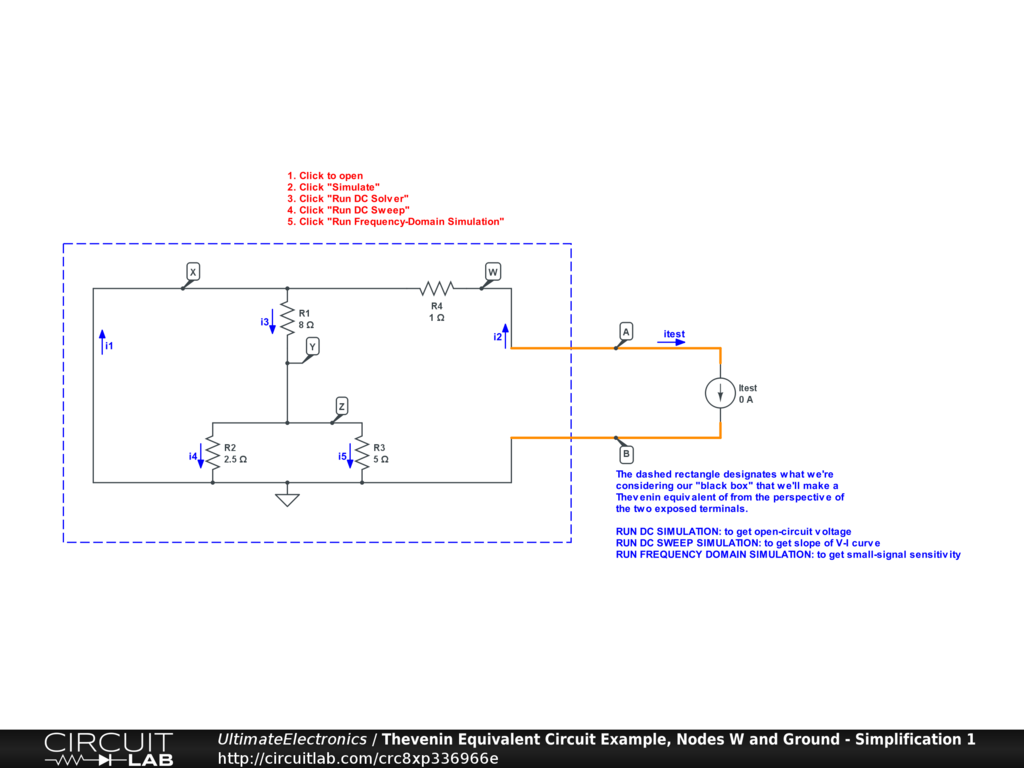Interactive Exercise Click to open and simulate the circuit above.

Now, we can simplify the circuit by inspection. The combination of $R_1 + R_2 // R_3$ is in parallel with a short-circuit wire which came from voltage source V1. Any nonzero resistance in parallel with a zero resistance wire will always have zero current through the finite resistance. The current will always follow the zero-resistance path instead. As a result, we can actually delete R1, R2, and R3 entirely: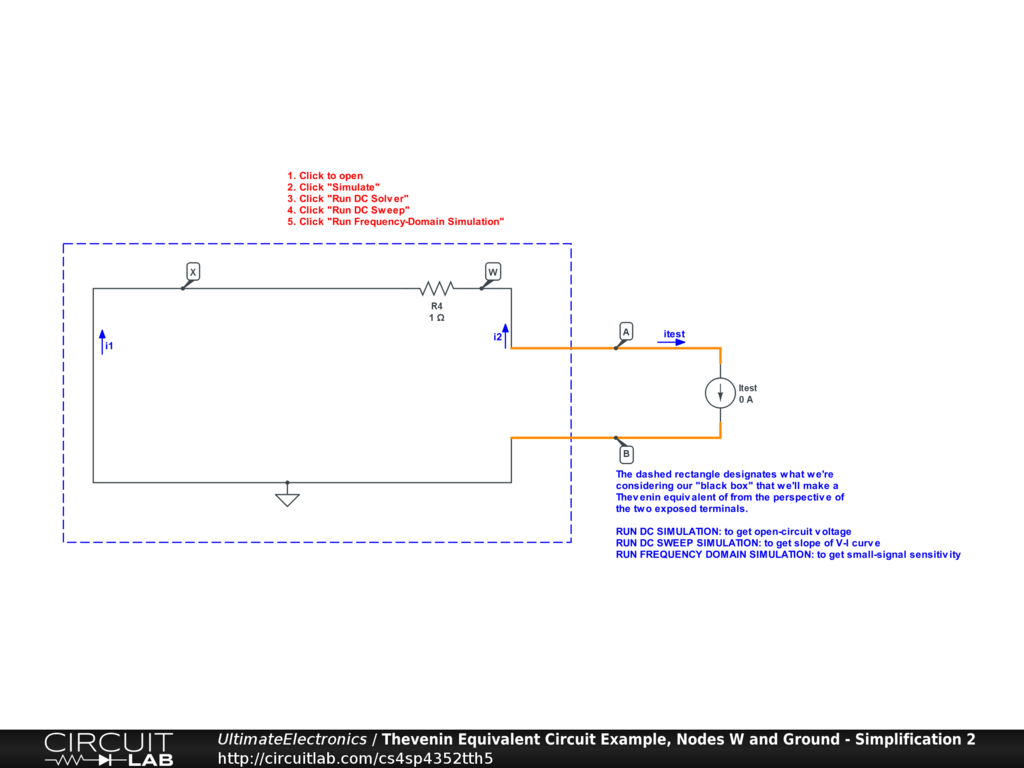Interactive Exercise Click to open and simulate the circuit above.

From this picture, it's obvious that our current source is just in series with a single resistor $R_4 = 1 \ \Omega$. As the current drawn by Itest increases, the voltage at node A will drop, $V_A = - i_{\text{test}} \cdot R_4$. Our equivalent resistance is just:

$$R_{\text{eq}} = 1 \ \Omega$$

For step 5: we'll construct the Thevenin equivalent circuit: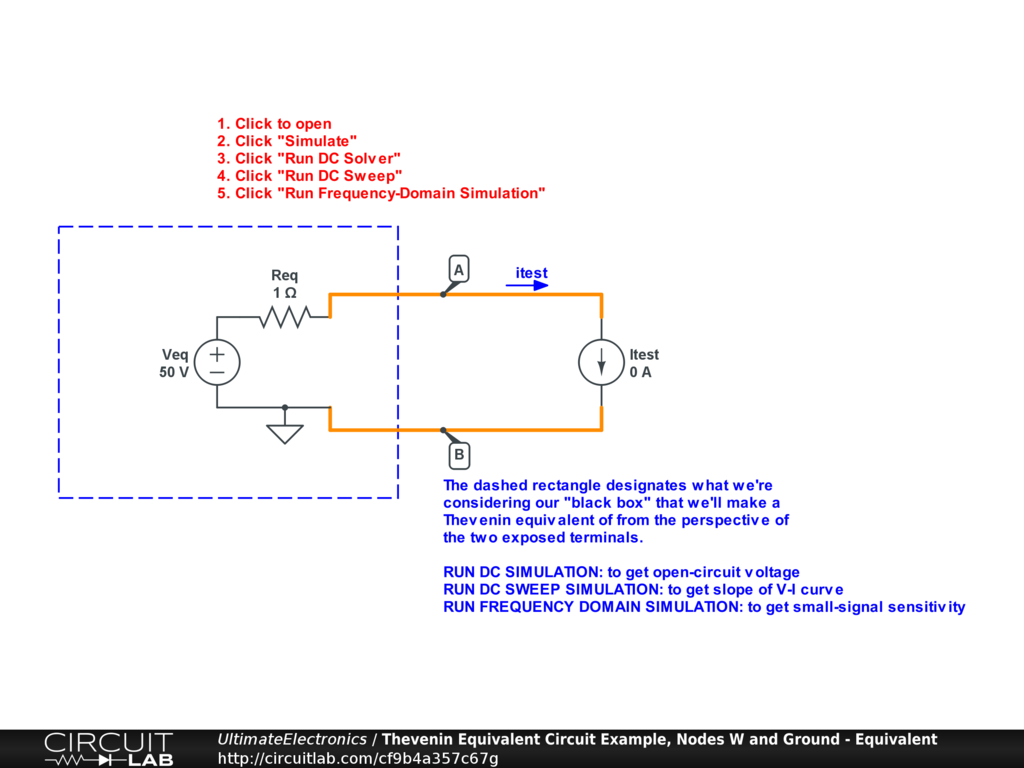Interactive Exercise If you open this circuit and run the DC or DC Sweep simulations, you'll see that this very simple Thevenin equivalent circuit behaves identically to the far more complicated original circuit above. That's the magic of the Thevenin equivalent circuit concept!

For nodes X and Z:

We can run through a similar process if a different pair of nodes were of interest: we'll just connect our test current source there, and repeat the same process.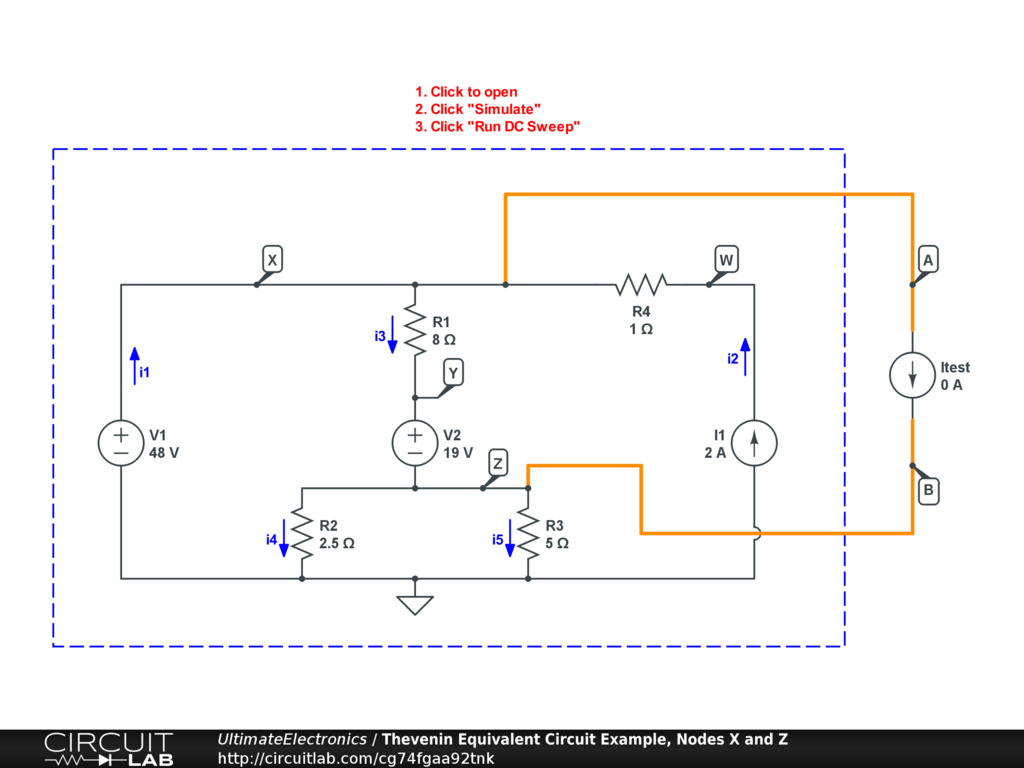Interactive Exercise Click to open and simulate the circuit above.

For step 1: we've identified internal nodes X and Z, and also called them externally nodes A and B.

For step 2: we've already solved this circuit in the Solving Circuit Systems and found that $V_X = 48$ and $V_Z = 5$, so $V_X - V_Z = 43$ and:

$$V_{\text{eq}} = 43 \ \text{V}$$

For step 3: we've added the test current source Itest.

For step 4: we can use superposition again, leaving only our test current source Itest. The voltage sources V1 and V2 are set to zero by being replaced by wires, and current source I1 is set to zero by being removed: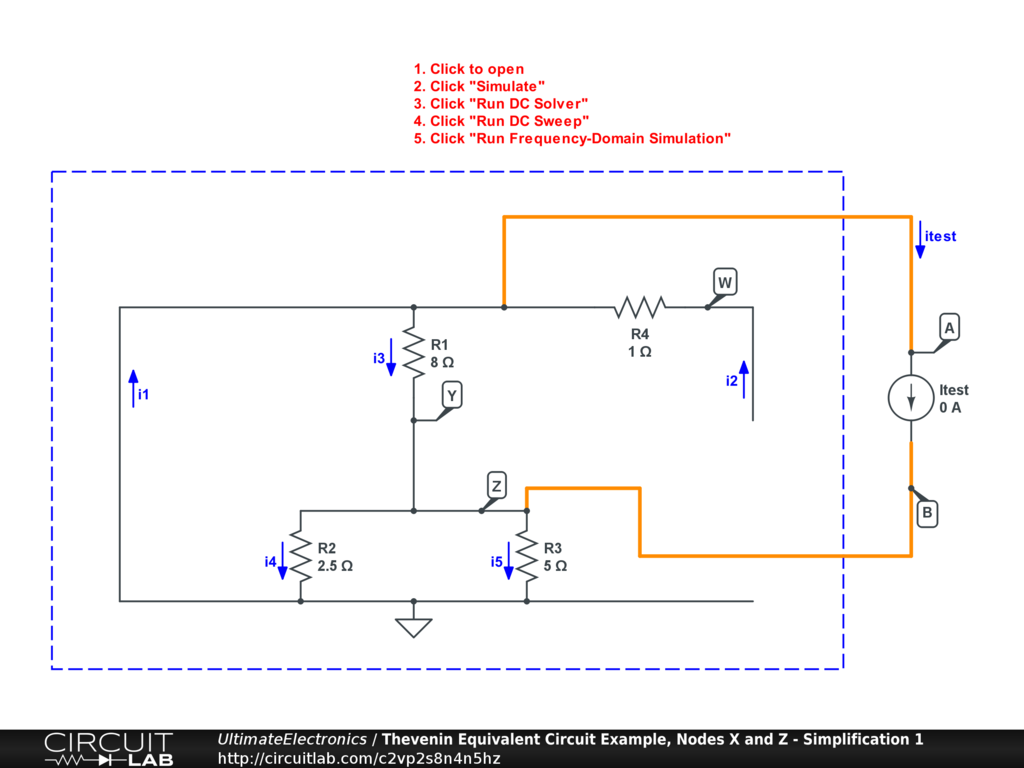Interactive Exercise Click to open and simulate the circuit above.

Now, we can make a few simplifications by inspection. First, we can delete resistor R4 since one end is hanging unconnected and it will have no effect on the circuit. Then, we can swing R1 around to be side-by-side with R2 and R3, as all three resistors are connected between the same pair of nodes: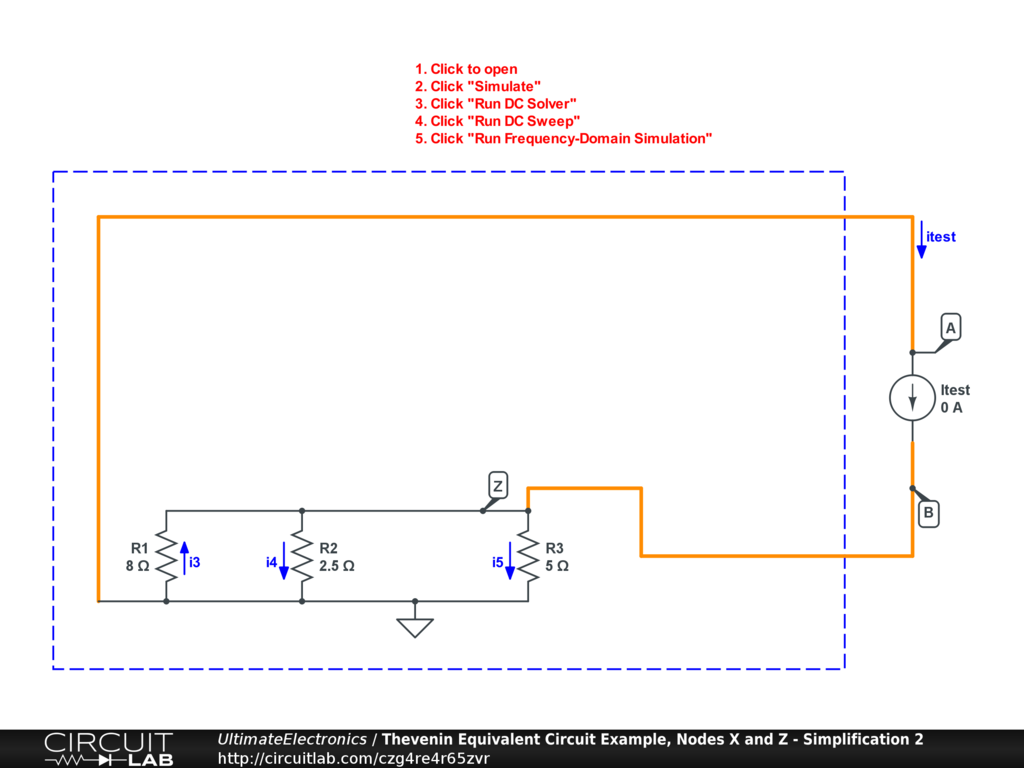Interactive Exercise Click to open and simulate the circuit above.

From this schematic, it's clear that the three resistors R1, R2, and R3 are in parallel, forming a parallel equivalent resistance of:

\begin{align} R_{\text{eq}} & = R_1 // R_2 // R_3 \\ & = \frac {1} { \frac{1} {R_1} + \frac{1} {R_2} + \frac{1} {R_3} } \\ & = \frac {1} { \frac{1} {8} + \frac{1} {2.5} + \frac{1} {5} } \\ & = \frac {1} { \frac{5} {40} + \frac {16} {40} + \frac {8} {40} } \\ R_{\text{eq}} & = \frac {40} {29} \ \Omega \\ R_{\text{eq}} & \approx 1.379 \ \Omega \end{align}

One of our nodes A is now a ground node, fixing $V_A = 0$ in this version of the superposition. Note that as Itest increases in the direction drawn, the voltage at node B rises. The voltage difference $V_A - V_B$ therefore decreases as the current Itest rises, as expected. This negative slope is still the equivalent resistance.

For step 5: we'll construct the Thevenin equivalent circuit: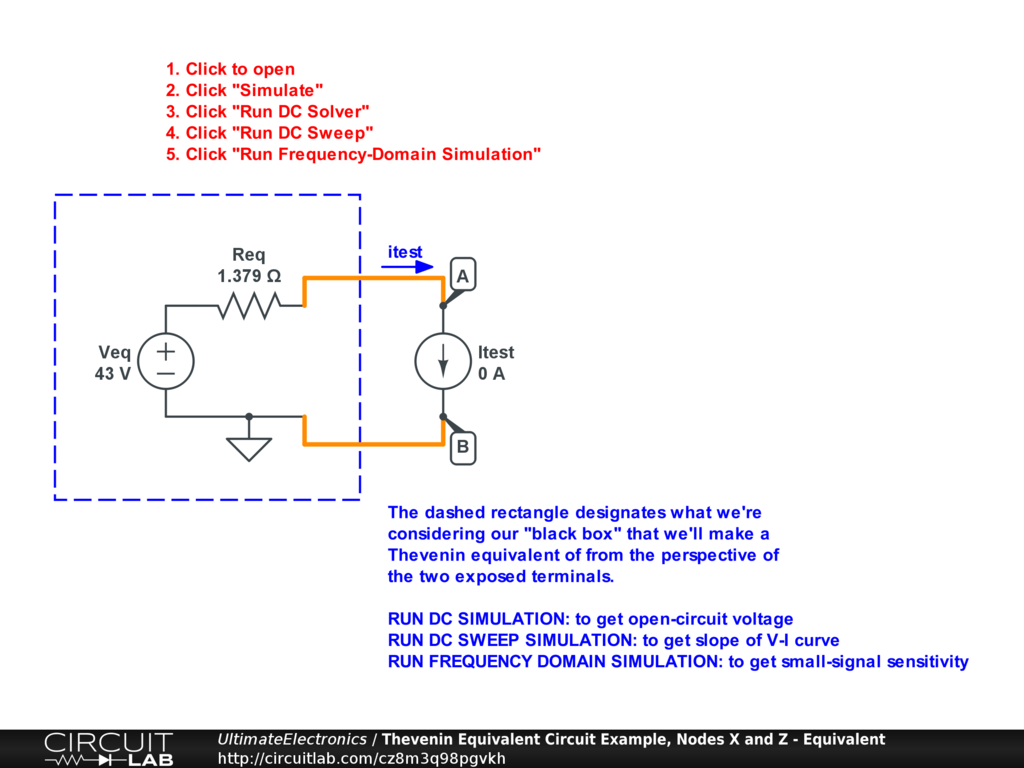Interactive Exercise Again, if you open this circuit and run the DC or DC Sweep simulations, you'll see that this Thevenin equivalent again performs identically to the more complicated version we started with.

Finding the Thevenin Equivalent Circuit by Simulation

The examples above illustrated how to solve for the Thevenin equivalent voltage and resistance by hand, and by using a DC Sweep simulation over the test current value to measure the slope of the V-I curve.

In general, this simulation process is:

1. Add a test current source. Set it to 0 current so that the DC solution will still reflect the open-circuit value.
2. Run the DC simulation. Measure the voltage difference across the test current source $\text{V(A)} - \text{V(B)}$. This is the open-circuit voltage.
3. Run the DC Sweep simulation. Vary the test current $\text{Itest.I}$ as your independent variable on the x-axis. Plot the voltage difference $\text{V(A)} - \text{V(B)}$ across the test current source on the y-axis. Graphically determine the slope of the voltage-current line. This slope (negative!) is your equivalent resistance.

This process works, but involves estimating a slope from a graphed line. There's a better process that works by using the Frequency Domain simulation mode of CircuitLab. While we aren't looking at any frequency-dependent components yet, the frequency domain simulation essentially determines how sensitive various currents and voltages are to a small change in any particular source. The notion of determining the slope of one circuit variable with respect to changes in another is built in to Frequency Domain simulation mode. For this process:

1. Add a test current source. Set it to 0 current so that the DC solution will still reflect the open-circuit value.
2. Run the DC simulation. Measure the voltage difference across the test current source $\text{V(A)} - \text{V(B)}$. This is the open-circuit voltage.
3. Run the Frequency Domain simulation. Set the test current source $\text{Itest}$ as your input. Plot the real number component of the voltage difference $\text{REAL(V(A)} - \text{V(B))}$ across the test current source. The value plotted will be a flat horizontal line regardless of frequency if there are no frequency-dependent components present. The number shown here is the negative equivalent resistance. It shows that, for a small increase $d i_{\text{test}}$, there is a small decrease in voltage $d V_{A B}$. The equivalent resistance is $$R_{\text{eq}} = \frac {d V_{A B}} {d i_{\text{test}}}$$ In CircuitLab's frequency domain simulation, the default source magnitude of the input is 1, so this will show you $-R_{\text{eq}}$ directly.

You can quickly test this by clicking any of the circuits (original or Thevenin equivalent) with a test current source above, then clicking "Simulate," "Frequency Domain," and finally "Run Frequency Domain Simulation."

• For the node W to ground example, you'll see a line plotted at -1, indicating that $R_{\text{eq}} = 1 \ \Omega$.
• For the node X to Z example, you'll see a line plotted at -1.379, indicating that $R_{\text{eq}} = 1.379 \ \Omega$.

(Click "Export Plot CSV" if you need more digits of precision!) These equivalent resistances exactly match the ones we found when reducing the problem by hand.

The benefit of learning how to solve Thevenin equivalent circuits by simulation is that the simulator is able to tackle problems of much higher complexity than you may wish to solve by hand, especially once we add active components like transistors and op-amps.

Nonlinear Thevenin Equivalent Circuits

Another significant reason to learn how to use the CircuitLab simulator to find Thevenin equivalent circuits is that it can also easily create linearized models of nonlinear circuits, including circuits with transistors, diodes, dependent sources, arbitrary behavioral sources, and more.

The equivalent circuit you find will only be valid in a region around the point of linearization. See the Algebraic Approximations for more on determining how to make these simplifications by hand, and when they're valid and useful.

Here's an example with a nonlinear circuit including an LED which we referenced during our discussion of power and efficiency: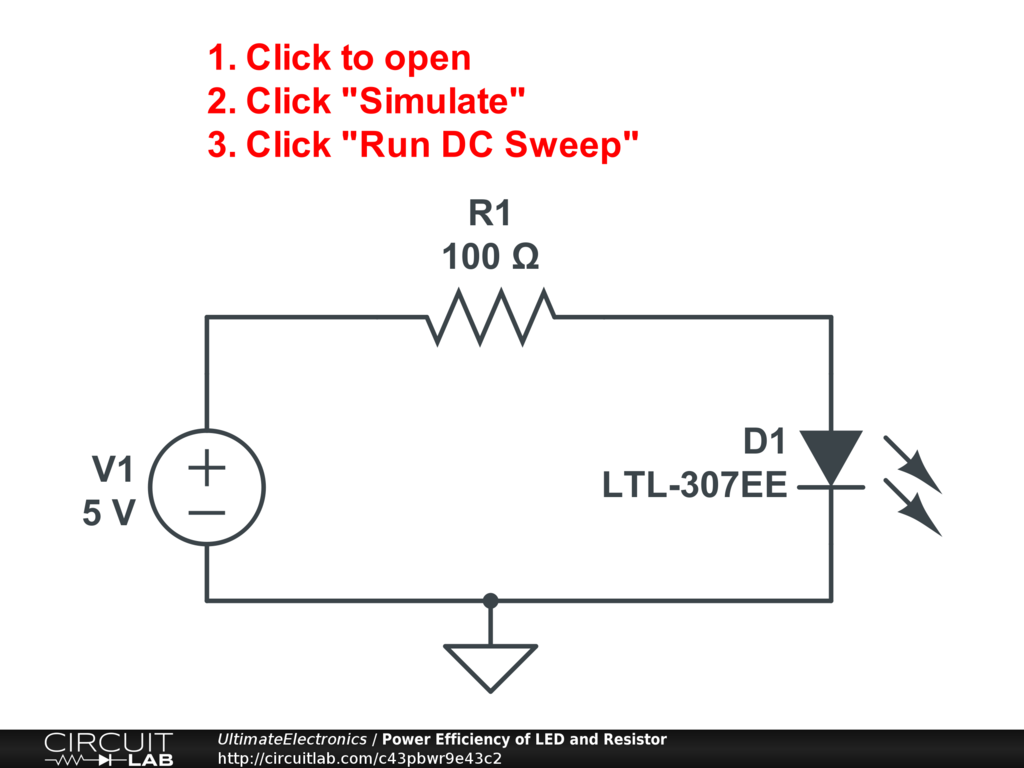Interactive Exercise Click to open and simulate the efficiency of the LED and resistor circuit above.

Let's suppose that we might connect other devices in parallel with the LED, and we want to determine roughly what the circuit's behavior will be for different loads. To do that, we can connect our test current source to the nodes of interest: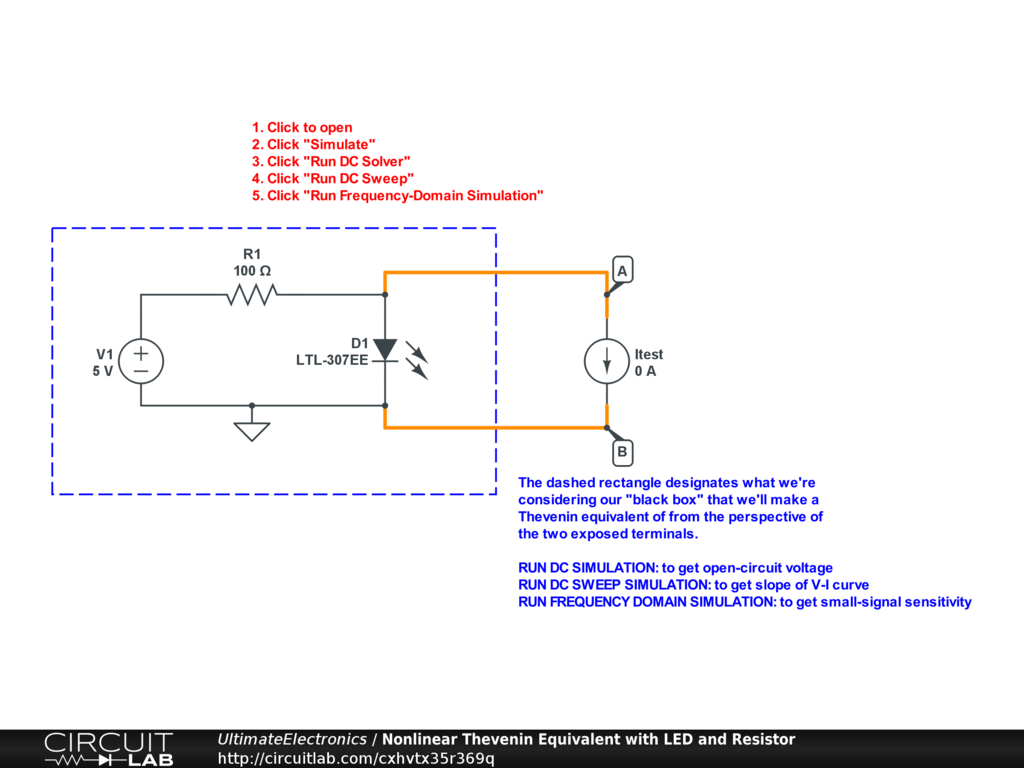Interactive Exercise Click the circuit and run the DC Sweep simulation to see both the nonlinear curve of behavior, overlayed with a plot of the Thevenin equivalent linearization.

As specified above, we've used the DC simulation to measure the open-circuit voltage as $V_{\text{eq}} = 2.138 \ \text{V}$, and we've used the frequency domain simulation to measure the slope as $R_{\text{eq}} = 9.786 \ \Omega$. Note that this slope is linearized around the DC simulation point: i.e. linearized around $i_{\text{test}} = 0$.

Because this is a nonlinear circuit, the approximation is only good in the region near the origin. The real V-I curve is not a line; it has a big bend in it visible on the DC Sweep simulation! Conceptually:

• For negative values of $i_{\text{test}} < 0$, the test current source is effectively supplying more current to the LED, which causes only a gentle rise in its operating voltage.
• But when $i_{\text{test}} > 0$, the test current begins to "steal" current from the LED, which soon turns off and becomes effectively open circuit, leaving resistor R1 to dominate.

If, for example, we change the value of V1 to be 10 volts instead of 5, we'll have a different operating point and this will change the value of both $V_{\text{eq}}$ and $R_{\text{eq}}$. In comparison, in a purely linear black-box circuit, changing any individual source might change $V_{\text{eq}}$, but would never change $R_{\text{eq}}$. As an exercise, see if you can compute the Thevenin equivalent circuit when V1 is set to 10 volts.

In conclusion, the technique of using the DC and Frequency Domain simulations to quickly compute the Thevenin equivalent circuit works for both linear and nonlinear circuits.

Norton Equivalent Circuits

From the perspective of outside terminals, any series combination of an ideal voltage source plus resistor (i.e. a Thevenin equivalent circuit) can be transformed into a parallel combination of an ideal current source plus resistor (called a Norton equivalent circuit).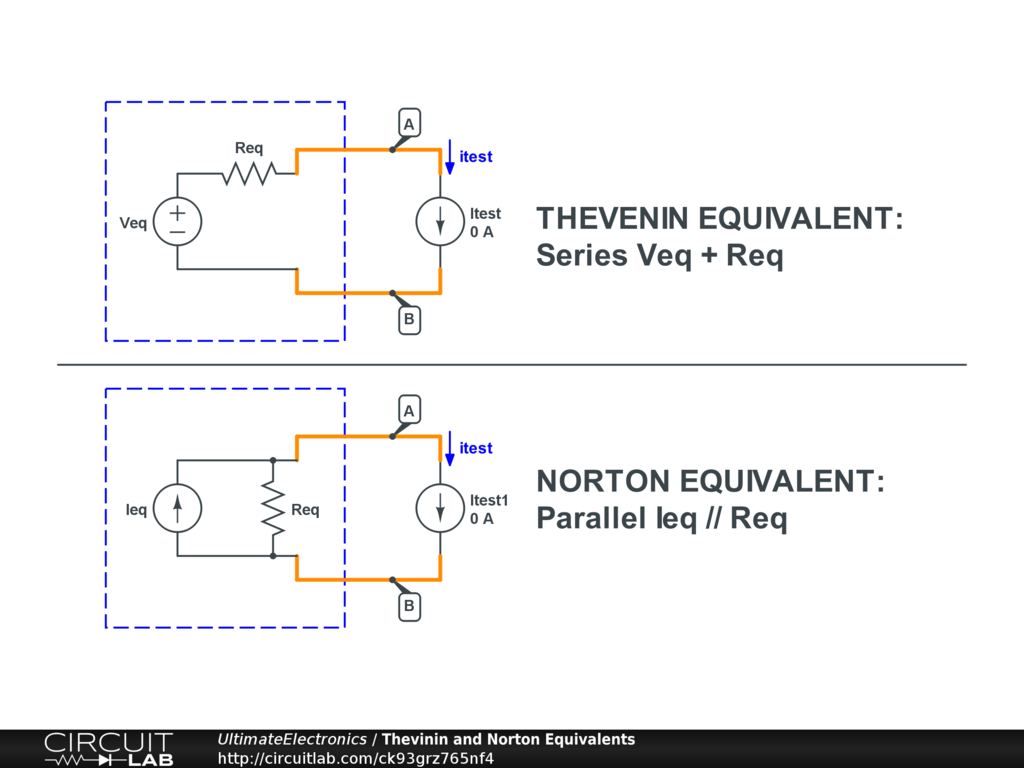For the Thevenin equivalent circuit, the open-circuit voltage is clearly $V_{\text{eq}}$. If we applied the Thevenin equivalent process to itself, we'd use superposition to turn off Veq and turn it into a wire, and the result would be that $R_{\text{eq}}$ would of course be the equivalent resistance.

For the Norton equivalent circuit, the open-circuit voltage is determined by the voltage drop across the resistor. Because at open circuit all the current $I_{\text{eq}}$ flows through the resistor, the open-circuit voltage is $I_{\text{eq}} \cdot R_{\text{eq}}$. Similarly, if we went to find the equivalent resistance by superposition, we'd just turn off the internal current source by making it an open circuit, leaving just the resistor, so again, $R_{\text{eq}}$ is the equivalent resistance.

These two simple circuits are equivalent if we let:

$$I_{\text{eq}} = \frac {V_{\text{eq}}} {R_{\text{eq}}}$$

Sometimes, it's more useful or convenient to think about parallel elements than about series ones. In these cases, we can just transform the Thevenin equivalent circuit into a Norton equivalent, or vice versa. From the perspective of the external test current source, the two are indistinguishable.

For a numerical example, the two circuits in these dashed rectangles are identical: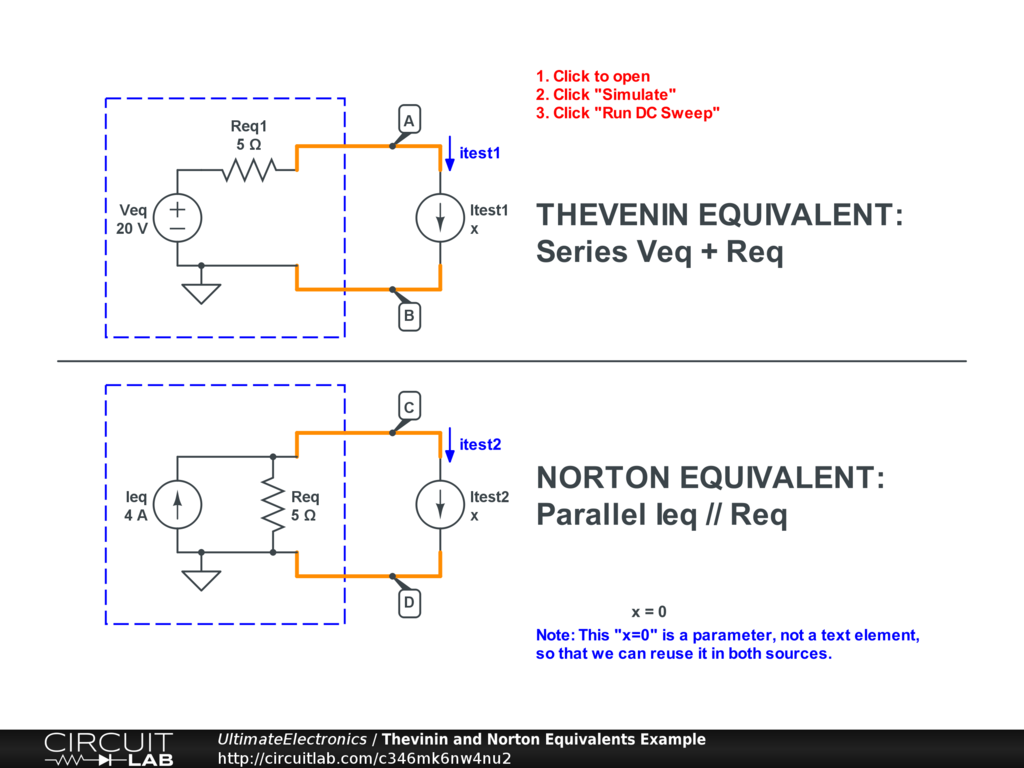Interactive Exercise Click the circuit, click "Simulate", and "Run DC Sweep" to see that the two V-I curves are completely overlapping each other, indicating that the external voltage difference of each equivalent circuit is the same for each value of test current.

What's Next

In the next section, Maximum Power Transfer and Impedance Matching, we'll show how interconnecting parts of circuits like those modeled by these equivalent circuits leads to consequences for transferring power and signals within a system.